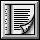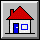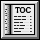5.3 Class COMPARABLE`indexing`
```description: "Objects that may be compared %
%according to a total order relation";
note: "The basic operation is < (less than); others %
%are defined in terms of this operation and is_equal."```
`deferred class interface`
`COMPARABLE`
`feature -- Comparison`
```infix "<" (other: like Current): BOOLEAN
-- Is current object less than other?
require
other_exists: other /= Void
deferred
ensure
asymmetric: Result implies not (other < Current)```
```infix "<=" (other: like Current): BOOLEAN
-- Is current object less than or equal to other?
require
other_exists: other /= Void
ensure
definition: Result = (Current < other) or is_equal (other);```
```infix ">=" (other: like Current): BOOLEAN
-- Is current object greater than or equal to other?
require
other_exists: other /= Void
ensure
definition: Result = (other <= Current)```
```infix ">" (other: like Current): BOOLEAN
-- Is current object greater than other?
require
other_exists: other /= Void
ensure
definition: Result = (other < Current)```
```is_equal (other: like Current): BOOLEAN
-- Is other attached to an object considered equal
-- to current object?
-- (Redefined from GENERAL.)
require
other_not_void: other /= Void
ensure
symmetric: Result implies other.is_equal (Current);
consistent: standard_is_equal (other) implies Result;
trichotomy: Result = (not (Current < other) and not (other < Current))```
```max (other: like Current): like Current
-- The greater of current object and other
require
other_exists: other /= Void
ensure
current_if_not_smaller: (Current >= other) implies (Result = Current)
other_if_smaller: (Current < other) implies (Result = other)```
```min (other: like Current): like Current
-- The smaller of current object and other
require
other_exists: other /= Void
ensure
current_if_not_greater: (Current <= other) implies (Result = Current)
other_if_greater: (Current > other) implies (Result = other)```
```three_way_comparison (other: like Current): INTEGER)
-- If current object equal to other, 0; if smaller,
-- -1; if greater, 1.
require
other_exists: other /= Void
ensure
equal_zero: (Result = 0) = is_equal (other);
smaller_negative: (Result = -1) = (Current < other);
greater_positive: (Result = 1) = (Current > other)```
`invariant`
`irreflexive_comparison: not (Current < Current)`
`end`

 Copyright © 1995, Nonprofit International Consortium for Eiffel mailto:nice@atlanta.twr.com Last Updated: 26 October 1997